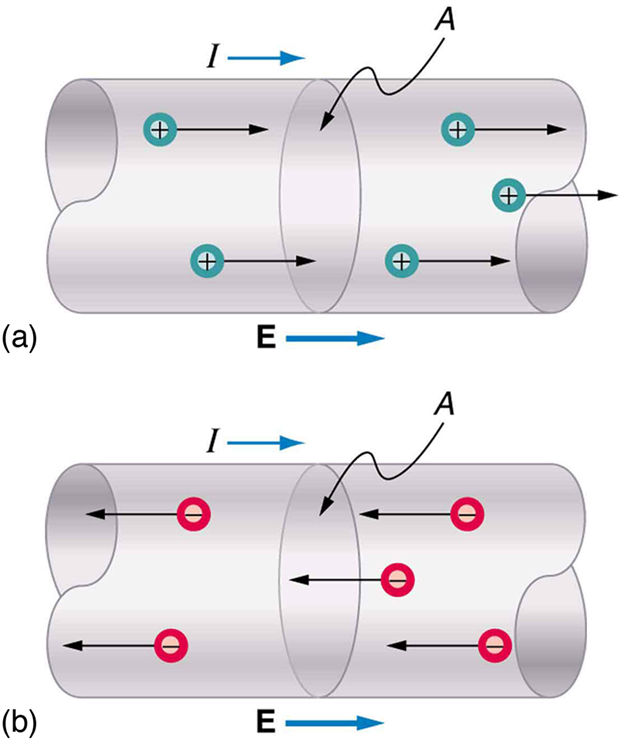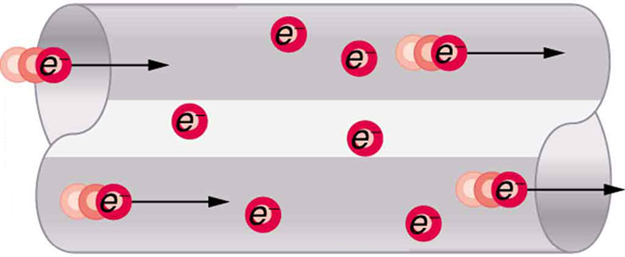# 20.1 Current  (Page 3/10)

 Page 3 / 10Current I size 12{I } {} is the rate at which charge moves through an area A , such as the cross-section of a wire. Conventional current is defined to move in the direction of the electric field. (a) Positive charges move in the direction of the electric field and the same direction as conventional current. (b) Negative charges move in the direction opposite to the electric field. Conventional current is in the direction opposite to the movement of negative charge. The flow of electrons is sometimes referred to as electronic flow.

## Calculating the number of electrons that move through a calculator

If the 0.300-mA current through the calculator mentioned in the [link] example is carried by electrons, how many electrons per second pass through it?

Strategy

The current calculated in the previous example was defined for the flow of positive charge. For electrons, the magnitude is the same, but the sign is opposite, ${I}_{\text{electrons}}=-0.300×{\text{10}}^{-3\phantom{\rule{0.25em}{0ex}}}\text{C/s}$ .Since each electron $\left({e}^{-}\right)$ has a charge of $–1\text{.}\text{60}×{\text{10}}^{-\text{19}}\phantom{\rule{0.25em}{0ex}}\text{C}$ , we can convert the current in coulombs per second to electrons per second.

Solution

Starting with the definition of current, we have

${I}_{\text{electrons}}=\frac{\text{Δ}{Q}_{\text{electrons}}}{\text{Δ}t}=\frac{–0\text{.}\text{300}×{\text{10}}^{-3}\phantom{\rule{0.25em}{0ex}}\text{C}}{\text{s}}\text{.}$

We divide this by the charge per electron, so that

$\begin{array}{lll}\frac{{e}^{\text{–}}}{\text{s}}& =& \frac{–0\text{.}\text{300}×{\text{10}}^{–3}\phantom{\rule{0.25em}{0ex}}\text{C}}{\text{s}}×\frac{\text{1}\phantom{\rule{0.25em}{0ex}}{e}^{\text{–}}}{–1\text{.60}×{\text{10}}^{-\text{19}}\phantom{\rule{0.25em}{0ex}}\text{C}}\\ & =& \text{1.88}×{\text{10}}^{\text{15}}\phantom{\rule{0.25em}{0ex}}\frac{{e}^{\text{–}}}{\text{s}}\text{.}\end{array}$

Discussion

There are so many charged particles moving, even in small currents, that individual charges are not noticed, just as individual water molecules are not noticed in water flow. Even more amazing is that they do not always keep moving forward like soldiers in a parade. Rather they are like a crowd of people with movement in different directions but a general trend to move forward. There are lots of collisions with atoms in the metal wire and, of course, with other electrons.

## Drift velocity

Electrical signals are known to move very rapidly. Telephone conversations carried by currents in wires cover large distances without noticeable delays. Lights come on as soon as a switch is flicked. Most electrical signals carried by currents travel at speeds on the order of ${\text{10}}^{8}\phantom{\rule{0.25em}{0ex}}\text{m/s}$ , a significant fraction of the speed of light. Interestingly, the individual charges that make up the current move much more slowly on average, typically drifting at speeds on the order of ${\text{10}}^{-4}\phantom{\rule{0.25em}{0ex}}\text{m/s}$ . How do we reconcile these two speeds, and what does it tell us about standard conductors?

The high speed of electrical signals results from the fact that the force between charges acts rapidly at a distance. Thus, when a free charge is forced into a wire, as in [link] , the incoming charge pushes other charges ahead of it, which in turn push on charges farther down the line. The density of charge in a system cannot easily be increased, and so the signal is passed on rapidly. The resulting electrical shock wave moves through the system at nearly the speed of light. To be precise, this rapidly moving signal or shock wave is a rapidly propagating change in electric field.When charged particles are forced into this volume of a conductor, an equal number are quickly forced to leave. The repulsion between like charges makes it difficult to increase the number of charges in a volume. Thus, as one charge enters, another leaves almost immediately, carrying the signal rapidly forward.

Suppose a speck of dust in an electrostatic precipitator has 1.0000×1012 protons in it and has a net charge of –5.00 nC (a very large charge for a small speck). How many electrons does it have?
how would I work this problem
Alexia
how can you have not an integer number of protons? If, on the other hand it supposed to be 1e12, then 1.6e-19C/proton • 1e12 protons=1.6e-7 C is the charge of the protons in the speck, so the difference between this and 5e-9C is made up by electrons
Igor
what is angular velocity
Why does earth exert only a tiny downward pull?
hello
Islam
Why is light bright?
an 8.0 capacitor is connected by to the terminals of 60Hz whoes rms voltage is 150v. a.find the capacity reactance and rms to the circuit
thanks so much. i undersooth well
what is physics
is the study of matter in relation to energy
Kintu
a submersible pump is dropped a borehole and hits the level of water at the bottom of the borehole 5 seconds later.determine the level of water in the borehole
what is power?
power P = Work done per second W/ t. It means the more power, the stronger machine
Sphere
e.g. heart Uses 2 W per beat.
Rohit
A spherica, concave shaving mirror has a radius of curvature of 32 cm .what is the magnification of a persons face. when it is 12cm to the left of the vertex of the mirror
did you solve?
Shii
1.75cm
Ridwan
my name is Abu m.konnek I am a student of a electrical engineer and I want you to help me
Abu
the magnification k = f/(f-d) with focus f = R/2 =16 cm; d =12 cm k = 16/4 =4
Sphere
what do we call velocity
Kings
A weather vane is some sort of directional arrow parallel to the ground that may rotate freely in a horizontal plane. A typical weather vane has a large cross-sectional area perpendicular to the direction the arrow is pointing, like a “One Way” street sign. The purpose of the weather vane is to indicate the direction of the wind. As wind blows pa
hi
Godfred
Godfred
If a prism is fully imersed in water then the ray of light will normally dispersed or their is any difference?
the same behavior thru the prism out or in water bud abbot
Ju
If this will experimented with a hollow(vaccum) prism in water then what will be result ?
Anurag
What was the previous far point of a patient who had laser correction that reduced the power of her eye by 7.00 D, producing a normal distant vision power of 50.0 D for her?
What is the far point of a person whose eyes have a relaxed power of 50.5 D?
Jaydie
What is the far point of a person whose eyes have a relaxed power of 50.5 D?
Jaydie
A young woman with normal distant vision has a 10.0% ability to accommodate (that is, increase) the power of her eyes. What is the closest object she can see clearly?
Jaydie
29/20 ? maybes
Ju
In what ways does physics affect the society both positively or negativelyByByBy OpenStaxBy OpenStaxBy Carly AllenBy Brooke DelaneyBy Madison ChristianBy Richley CrapoBy John GabrieliByBy Heather McAvoyBy Marion Cabalfin